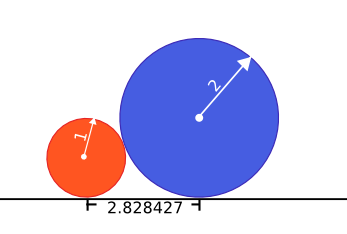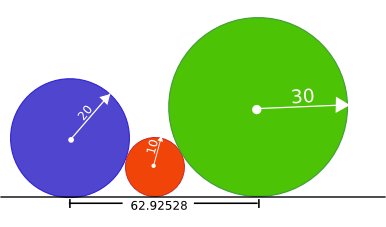Tuesday, May 21, 2013

Problem Statement

NOTE: This problem statement contains images that may not display properly if viewed outside of the applet.

Everybody loves geometry, so here is a geometry problem. You have a few marbles of possibly different sizes. You are given a vector <int> radius that describes the marbles: each element of radius is the radius of one of your marbles.

You want to place all marbles onto a straight line that is drawn on the table. Clearly, this makes the problem two-dimensional: we can just view the marbles as circles that will all be touching the line from above. Of course, the marbles cannot overlap, so in our problem no two circles are allowed to overlap. Note that you may place the marbles onto the line in any order, you do not have to preserve the order in which they are given in radius.

Additionally, you want to pack the bottoms of the marbles as close together as possible. More precisely: For each marble consider the point where it touches the line. Compute and return the smallest possible distance between the farthest two of those points. (That is, if you imagine the line as going from the left to the right, your task is to minimize the distance between the leftmost and the rightmost of the points where the circles touch the line.)

Definition

 Class: MarblePositioning Method: totalWidth Parameters: vector Returns: double Method signature: double totalWidth(vector radius) (be sure your method is public)

Notes

- The returned values must have an absolute or relative error less than 1e-9.

Constraints

- radius will contain between 2 and 8 elements, inclusive.
- Each element of radius will be between 1 and 1000000000 (10^9), inclusive.

Examples

0)

 `{1, 2}`
`Returns: 2.8284271247461903`
 One of the best ways to place the marbles is the following one:1)

 `{7,7,7}`
`Returns: 28.0`
2)

 `{10, 20, 30}`
`Returns: 62.92528739883945`3)

 `{100, 100,11,11,25}`
`Returns: 200.0`4)

 `{1,999950884,1}`
`Returns: 63246.0`

This problem statement is the exclusive and proprietary property of TopCoder, Inc. Any unauthorized use or reproduction of this information without the prior written consent of TopCoder, Inc. is strictly prohibited. (c)2003, TopCoder, Inc. All rights reserved.

[Thoughts]

``````1:  #include <string>
2:  #include <vector>
3:  #include <cmath>
4:  using namespace std;
5:  class MarblePositioning
6:  {
7:       struct Circle
8:       {
9:            double x;
10:            int r;
11:       };
12:  public:
13:       double GetXOfCenter(vector<Circle>& arrange, Circle& added);
14:       double GetCurrentLength(vector<Circle>& c);
15:       void GetMinArrangement(vector<Circle>& circles, vector<Circle>& arrange, vector<bool>& visited, double& minLen);
17:  };
18:  // Get the X coordinate of center of the circle.
19:  // Here, need go through all the circles instead of comparing with previous circle only, in case of circle overlap.
``````20:  double MarblePositioning::GetXOfCenter(vector<Circle>& arrange, Circle& added)
21:  {
22:       double x=0;
23:       for(int i =0; i<arrange.size(); i++)
24:       {
25:            // sqrt( (ra+rb)^2 - (ra-rb)^2)
26:            double temp = arrange[i].x + 2* sqrt(arrange[i].r*added.r);
27:            if(temp>x) x= temp;
28:       }
29:       return x;
30:  }  ``````
31:  // Get t``````he length of current circle queue
32:  double MarblePositioning::GetCurrentLength(vector<Circle>& c)
33:  {
34:       if(c.size() ==0) return 0;
35:       return c[c.size()-1].x - c.x;
36:  }
37:  void MarblePositioning::GetMinArrangement(vector<Circle>& circles, vector<Circle>& arrange, vector<bool>& visited, double& minLen)
38:  {
39:       if(GetCurrentLength(arrange) > minLen) return; // pruning all non-optimization branch
40:       if(circles.size() == arrange.size())
41:       {
42:            double newLen = GetCurrentLength(arrange);
43:            if(newLen < minLen) minLen = newLen;
44:            return;
45:       }
46:       for(int i =0; i < circles.size(); i++)
47:       {
48:            if(visited[i]) continue;
49:            circles[i].x = GetXOfCenter(arrange, circles[i]);
50:            arrange.push_back(circles[i]);
51:            visited[i] = true;
52:            GetMinArrangement(circles, arrange, visited, minLen);
53:            arrange.pop_back();
54:            visited[i] = false;
55:            circles[i].x =0;
56:       }
57:  }
59:  {
61:       for(int i =0; i< radius.size(); i++)
62:       {
64:            sample[i].x = 0;
65:       }
66:       vector<Circle> arrange;
68:       double minLen = INT_MAX;
69:       GetMinArrangement(sample, arrange, visited, minLen);
70:       return minLen;
71:  }
``````

[Some Notes]
1. Function  GetMinArrangement  的 visited数组是不必要的，可以通过判断circle.x ==0来代替，这里完全是为了可读性。